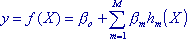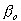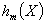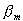## What are Multivariate Adaptive Regression Splines?

Multivariate Adaptive Regression Splines (MARS) is a technique to predict the values of unknown continuous dependent variables (outcome) with just a set of independent (predictor) variables.

MARS makes no starting guess about the functional relationship, such as linear, logarithmic, etc... between the dependent and independent variables, so it can also be considered a nonparametric regression procedure. Instead, MARS uses a set of coefficients and basis functions derived from the regression data to predict this relationship. In practice, this takes the approach of splitting the input space into ever smaller regions, each with its own regression equation. This also means MARS is less susceptible to problems caused by working with multiple variables, known as the “curse of higher dimensionality.”

## How do Multivariate Adaptive Regression Splines Work?

MARS creates a model with a two-step, forward and backward pass approach, just like in recursive partitioning trees. The forward pass starts with finding the mean of the response values (intercept term) and then repeatedly adding basis functions to the model. The basis functions are added in pairs, using a greedy algorithm approach. The backward pass then goes back through these pairs, deleting the least effective functions using generalized cross validation until only the “best fit” model is left.

The basic MARS model is defined as:Where:

X is a function of the predictor variables and their interactionsis the intercept parameterare the basis functionsis the weighted sum of all basis functions